# Velocity – Time Graphs

• Last Updated : 20 May, 2021

Anything that contains Kinetic energy is in some type of motion, whether it is one-dimensional, two-dimensional, rotational motion, etc. Kinetic energy is responsible for motion, and it is already known that the motion of any object happens because of some external force, the inertia present in the objects do not allow them to move or stop on their own, therefore, some external force is required to provide some kinetic energy to the object, and providing energy itself states that the object has some velocity. There can be several types of velocities an object in motion can have, and explaining the characteristic of velocity w.r.t time is easier graphically.

### Velocity of an object

The velocity of an object can be defined as the rate of change of displacement, or it can also be defined as the change in the object’s position according to a given frame of reference with respect to time. The velocity of an object is a vector quantity, which means. Velocity has both magnitude and direction to it and this is how it differs from speed, as the speed of an object does not contain direction.

Attention reader! All those who say programming isn't for kids, just haven't met the right mentors yet. Join the  Demo Class for First Step to Coding Course, specifically designed for students of class 8 to 12.

The students will get to learn more about the world of programming in these free classes which will definitely help them in making a wise career choice in the future.

Example 1: Tinkesh walks from his office to his home in 30 minutes and the displacement from the office to home is 2 kilometers. Find the value of the constant velocity at which Tinkesh is walking?

Solution:

It is known that the velocity of any person/object is the rate of change of its displacement.

Therefore, Velocity= displacement/Time

Given, displacement = 2km = 2000 meters

Time = 30 minutes = 1800 seconds.

Velocity = 2000/1800

Velocity= 1.11m/sec

Example 2: Two objects are moving with a speed 20m/sec and 40m/sec in order to reach the same destination which was 8000 meters away from the starting point of both the objects. Find the time taken by both objects.

Solution:

Time taken by the first object (T1)= displacement/Velocity

T1= 8000/20

T1= 400seconds

T1= 400/60= 20/3 minutes.

Time taken by the second object (T2) = 8000/40

T2= 200 seconds

T2= 200/60 minutes

T2= 10/3 minutes

### Velocity- Time Graphs

Velocity-Time graph is very important while learning about the motion of any object, the graph tells about the acceleration of the object. Depending upon the different types of velocities, for example, an object may have a constant velocity, increasing velocity, or decreasing velocity, it can easily be determined if the object is accelerating, decelerating, or not accelerating at all.

Case 1: Constant Velocity (No Acceleration)

When the Velocity of an object is the same through its motion, the initial and final velocity remains the same and the object will have some initial velocity and cannot have zero initial velocity, the slope of the velocity-time graph obtained is constant. The constant slope represents no increase or no decrease in the acceleration of the object. Hence, the object has zero acceleration in this case.

In the graph shown below, the x-axis represents time, the y-axis represents the velocity. The acceleration is zero and hence, the equations of Motion are not applicable here, the simple formula given above is used to find out the velocity of the object.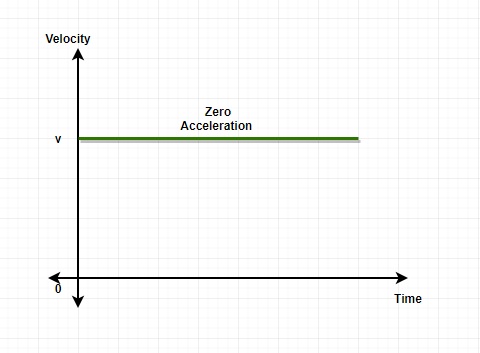Case 2: Increasing Velocity (Constant Acceleration)

When the velocity of an object is increasing throughout the motion, the final velocity becomes more than the initial velocity, the initial velocity, in this case, can be zero as well, since the object is constantly increasing its speed. The slope of the velocity-time graph obtained in this case is increasing in nature, the increasing slope tells that the body is in constant acceleration.

The x-axis on the graph represents time and the y-axis represents the velocity. The equations of motion are applicable in this to find out the parameters of the object in motion since the equations are allowed for object undergoing constant acceleration.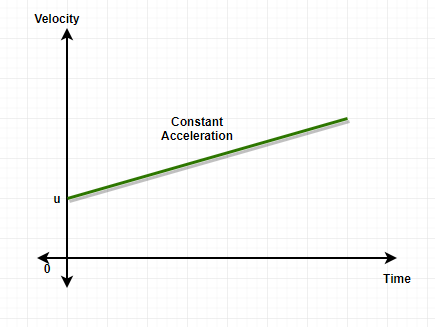Case 3: Increasing rate of change of velocity (Increasing acceleration)

When the rate of change of velocity is increasing throughout the motion, the final velocity will be much more than the initial velocity, The initial velocity in this case as well can be zero since the object’s acceleration is increasing every second at some rate. The slope of the velocity-time graph in this case will take the form of a curve, The curve in the slope tells that the body has increasing/decreasing acceleration.

The x-axis on the graph represents time and the y-axis represents velocity. The slope at any point of the graph will give the value of acceleration at that point.Note: Just like increasing velocity-time graph, there can be decreasing velocity-time graph where the slope is decreasing since the velocity is decreasing in nature, and just like the increasing acceleration graph, there exist graph for decreasing acceleration as well with a negative slope curve since the object is undergoing the rate of change of deceleration.

### Sample Problems

Question 1: Draw the graph for an object starting at a velocity of 20m/sec and the final velocity becomes 0 m/sec at T=10th second. The object is decelerating in nature.

The graph will have a negative slope since the object is deceleration in nature.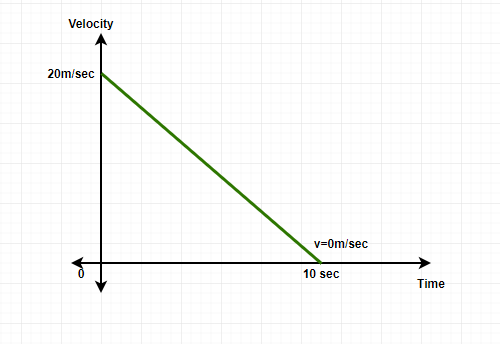Velocity-time graph

Question 2: An object with an initial velocity of 15m/sec is decelerating and ultimately coming to a stop at T= 20seconds, Find the Displacement covered by the object.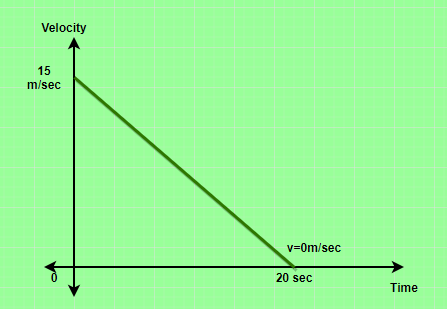Solution:

The displacement of the object can be found out by finding the area of the velocity-time graph.

The graph given forms a right angle triangle and the area of this right angle triangle will give the displacement covered by the object.

Area of the right angle triangle= Displacement of the object= 1/2× Base × Height

Displacement= 1/2 × 20× 15

Displacement = 150 meters.

Question 3: Find the Acceleration of the object which is constant in nature, The graph for the object’s velocity is given below.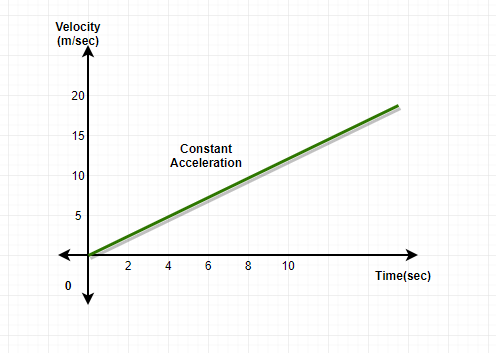The acceleration in the velocity-time graph is found out through the slope, the slope is the ratio of the value at y-axis and x-axis, solving this will easily give the value of acceleration of the object.

At time T= 8 sec, the value of the velocity of the object is 8 m/sec.

Hence, the slope of the graph at T= 10 sec will be,

Slope of the graph= Acceleration of the object = 8/10

Acceleration = 0.8 m/sec2

Question 4: Alakh decides to go on a short bike ride for 10 minutes. He was riding with a constant acceleration of 2 m/sec2, What is the find velocity of Alakh’s bike by the end of the ride?

Solution:

Applying first Equation of Motion to find out the velocity of Alakh’s bike,

v= u+ at

Here, the initial velocity (u)= 0 m/sec

Acceleration (a)= 2 m/sec2

Time = 10 minutes= 60 seconds

v= 0+ 60× 2

v= 120m/sec

My Personal Notes arrow_drop_up